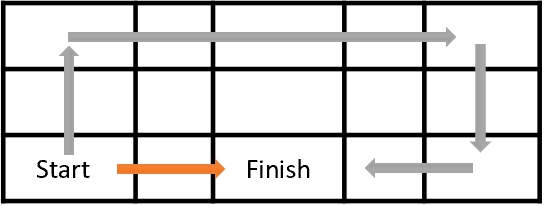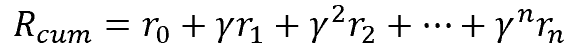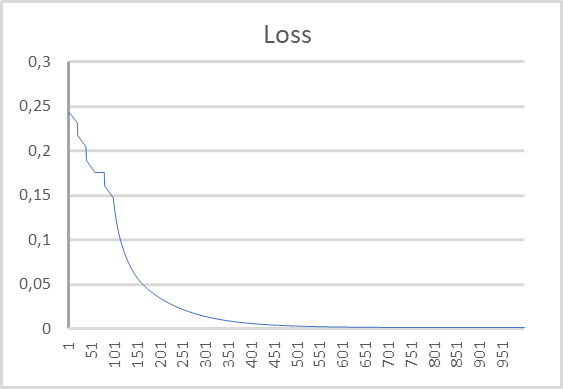# 神经网络变得轻松（第二十七部分）：深度 Q-学习（DQN）

### 内容

• 概述
• 1. Q-函数的概念
• 2. 深度 Q-学习
• 2.1. 体验回放
• 2.2. 使用目标网络
• 3. 利用 MQL5 实现
• 4. 测试
• 结束语
• 参考文献列表
• 本文中用到的程序

### 2. 深度 Q-学习#### 2.2. 使用目标网络

1. 为了训练代理者，我们运用神经网络。
2. 神经网络经过训练，可预测动作功用 Q-函数的期望值。
3. 为了令相邻状态之间的相关性最小化，学习过程使用记忆缓冲区，从中随机提取状态。
4. 为了预测 Q-函数的未来值，准备了第二个目标网络模型，它是训练模型的“冻结”副本。
5. 目标网络是周期性从已训练模型中复制权重矩阵来实现的。

### 3. 利用 MQL5 实现

1. 一笔盈利持仓获得与烛条主体大小相等的奖励（分析每根烛条的系统状态；我们处于从烛条开盘到收盘的位置）。
2. “场外观望”状态按照烛台实体的大小（烛台主体大小带负号表示亏损）作为惩罚。
3. 亏损持仓受到双倍烛台主体大小（亏损 + 利润损失）的惩罚。

`CNet                StudyNet;  CNet                TargetNet;      `

• Batch — 权重更新批量大小
• UpdateTarget — 在复制到“冻结”模型之前，预测未来 Q-函数值的已训练模型的权重矩阵的更新次数
• Iterations — 训练期间已训练模型更新的迭代总数
• DiscountFactor — 未来奖励折扣因子
`input int                  Batch =  100;  input int                  UpdateTarget = 20;  input int                  Iterations = 1000;  input double               DiscountFactor =   0.9;      `

`//---     float temp1, temp2;     if(!StudyNet.Load(FileName + ".nnw", dError, temp1, temp2, dtStudied, false) ||        !TargetNet.Load(FileName + ".nnw", dError, temp1, temp2, dtStudied, false))        return INIT_FAILED;      `

`   if(!StudyNet.GetLayerOutput(0, TempData))        return INIT_FAILED;     HistoryBars = TempData.Total() / 12;     StudyNet.getResults(TempData);     if(TempData.Total() != Actions)        return INIT_PARAMETERS_INCORRECT;      `

EA 初始化函数的其余部分保持不变。 附件中提供了其完整代码。

`void Train(void)    {  //---     MqlDateTime start_time;     TimeCurrent(start_time);     start_time.year -= StudyPeriod;     if(start_time.year <= 0)        start_time.year = 1900;     datetime st_time = StructToTime(start_time);  //---     int bars = CopyRates(Symb.Name(), TimeFrame, st_time, TimeCurrent(), Rates);     if(!RSI.BufferResize(bars) || !CCI.BufferResize(bars) || !ATR.BufferResize(bars) || !MACD.BufferResize(bars))       {        ExpertRemove();        return;       }     if(!ArraySetAsSeries(Rates, true))       {        ExpertRemove();        return;       }  //---     RSI.Refresh();     CCI.Refresh();     ATR.Refresh();     MACD.Refresh();      `

• total — 训练样本的大小
• use_target — 目标网络所用的标志，来预测未来奖励
`   int total = bars - (int)HistoryBars - 240;     bool use_target = false;      `

`   for(int iter = 0; (iter < Iterations && !IsStopped()); iter += UpdateTarget)       {        int i = 0;      `

`      for(int batch = 0; batch < Batch * UpdateTarget; batch++)          {           i = (int)((MathRand() * MathRand() / MathPow(32767, 2)) * (total));           State1.Clear();           State2.Clear();           int r = i + (int)HistoryBars;           if(r > bars)              continue;      `

`         for(int b = 0; b < (int)HistoryBars; b++)             {              int bar_t = r - b;              float open = (float)Rates[bar_t].open;              TimeToStruct(Rates[bar_t].time, sTime);              float rsi = (float)RSI.Main(bar_t);              float cci = (float)CCI.Main(bar_t);              float atr = (float)ATR.Main(bar_t);              float macd = (float)MACD.Main(bar_t);              float sign = (float)MACD.Signal(bar_t);              if(rsi == EMPTY_VALUE || cci == EMPTY_VALUE || atr == EMPTY_VALUE || macd == EMPTY_VALUE || sign == EMPTY_VALUE)                 continue;              //---              if(!State1.Add((float)Rates[bar_t].close - open) || !State1.Add((float)Rates[bar_t].high - open) ||                 !State1.Add((float)Rates[bar_t].low - open) || !State1.Add((float)Rates[bar_t].tick_volume / 1000.0f) ||                 !State1.Add(sTime.hour) || !State1.Add(sTime.day_of_week) || !State1.Add(sTime.mon) ||                 !State1.Add(rsi) || !State1.Add(cci) || !State1.Add(atr) || !State1.Add(macd) || !State1.Add(sign))                 break;              if(!use_target)                 continue;              //---              bar_t --;              open = (float)Rates[bar_t].open;              TimeToStruct(Rates[bar_t].time, sTime);              rsi = (float)RSI.Main(bar_t);              cci = (float)CCI.Main(bar_t);              atr = (float)ATR.Main(bar_t);              macd = (float)MACD.Main(bar_t);              sign = (float)MACD.Signal(bar_t);              if(rsi == EMPTY_VALUE || cci == EMPTY_VALUE || atr == EMPTY_VALUE || macd == EMPTY_VALUE || sign == EMPTY_VALUE)                 continue;              //---              if(!State2.Add((float)Rates[bar_t].close - open) || !State2.Add((float)Rates[bar_t].high - open) ||                 !State2.Add((float)Rates[bar_t].low - open) || !State2.Add((float)Rates[bar_t].tick_volume / 1000.0f) ||                 !State2.Add(sTime.hour) || !State2.Add(sTime.day_of_week) || !State2.Add(sTime.mon) ||                 !State2.Add(rsi) || !State2.Add(cci) || !State2.Add(atr) || !State2.Add(macd) || !State2.Add(sign))                 break;             }      `

`         if(IsStopped())             {              ExpertRemove();              return;             }           if(State1.Total() < (int)HistoryBars * 12 ||              (use_target && State2.Total() < (int)HistoryBars * 12))              continue;           if(!StudyNet.feedForward(GetPointer(State1), 12, true))              return;           if(use_target)             {              if(!TargetNet.feedForward(GetPointer(State2), 12, true))                 return;              TargetNet.getResults(TempData);             }      `

`         Rewards.Clear();           double reward = Rates[i - 1 + 240].close - Rates[i - 1 + 240].open;           if(reward >= 0)             {              if(!Rewards.Add((float)(reward + (use_target ? DiscountFactor * TempData.At(0) : 0))) ||                 !Rewards.Add((float)(-2 * (use_target ? reward + DiscountFactor * TempData.At(1) : 0)))                 ||                 !Rewards.Add((float)(-reward + (use_target ? DiscountFactor * TempData.At(TempData.Maximum(0, 3)) : 0))))                 return;             }           else              if(!Rewards.Add((float)(2 * reward + (use_target ? DiscountFactor * TempData.At(0) : 0))) ||                 !Rewards.Add((float)(-reward + (use_target ? DiscountFactor * TempData.At(1) : 0))) ||                 !Rewards.Add((float)(reward + (use_target ? DiscountFactor * TempData.At(TempData.Maximum(0, 3)) : 0))))                 return;      `

`         if(!StudyNet.backProp(GetPointer(Rewards)))              return;          }      `

`      if(!StudyNet.Save(FileName + ".nnw", StudyNet.getRecentAverageError(), 0, 0, Rates[i].time, false))           return;        float temp1, temp2;        if(!TargetNet.Load(FileName + ".nnw", dError, temp1, temp2, dtStudied, false))           return;        use_target = true;        PrintFormat("Iteration %d, loss %.5f", iter, StudyNet.getRecentAverageError());       }      `

`   Comment("");  //---     ExpertRemove();    }      `

### 4. 测试

1. 初始数据层，240 个元素（20 根蜡烛，每根蜡烛含 12 个神经元）。
2. 卷积层，输入数据窗口 24（2 根蜡烛），步长 12（1 根蜡烛），6 个滤波器输出。
3. 卷积层，输入数据窗口 2，步长 1，2 个过滤器。
4. 卷积层，输入数据窗口 3，步长 1，2 个过滤器。
5. 卷积层，输入数据窗口 3，步长 1，2 个过滤器。
6. 含有 1000 个元素的完全连接神经层。
7. 含有 1000 个元素的完全连接神经层。
8. 由 3 个元素组成的完全连接层（3 个操作的结果层）。### 参考文献列表

1. 运用深度强化学习玩转 Atari
2. 神经网络变得轻松（第二十五部分）：实践迁移学习
3. 神经网络变得轻松（第二十六部分）：强化学习

### 本文中用到的程序

# 名称 类型 说明
1 Q-learning.mq5 EA 训练模型的智能系统
2 NeuroNet.mqh 类库 创建神经网络模型的类库
3 NeuroNet.cl 代码库 创建神经网络模型的 OpenCL 程序代码库

MyFxtops迈投(www.myfxtops.com)-靠谱的外汇跟单社区，免费跟随高手做交易！